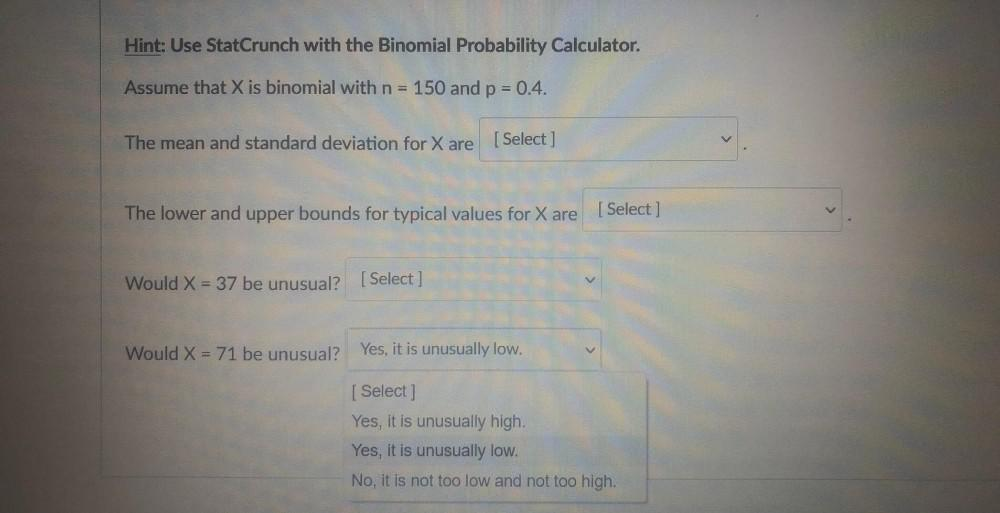Question:

# Hint: Use StatCrunch with the Binomial Probability Calculator. Assume that X is binomial with n = 150 and p = 0.4. The mean andHint: Use StatCrunch with the Binomial Probability Calculator. Assume that X is binomial with n = 150 and p = 0.4. The mean and standard deviation for X are [Select ] The lower and upper bounds for typical values for X are [Select] Would X = 37 be unusual? [Select ] Would X = 71 be unusual? Yes, it is unusually low. [ Select ] Yes, it is unusually high. Yes, it is unusually low. No, it is not too low and not too high.